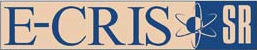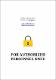Молимо вас користите овај идентификатор за цитирање или овај линк до ове ставке: `https://scidar.kg.ac.rs/handle/123456789/10077`
 Назив: Analytical solution for the problem of maximum exit velocity under Coulomb friction in gravity flow discharge chutes Аутори: Šalinić, SlavišaДатум издавања: 2010 Сажетак: In this paper, an analytical solution for the problem of finding profiles of gravity flow discharge chutes required to achieve maximum exit velocity under Coulomb friction is obtained by application of variational calculus. Themodel of a particle whichmoves down a rough curve in a uniform gravitational field is used to obtain a solution of the problem for various boundary conditions. The projection sign of the normal reaction force of the rough curve onto the normal to the curve and the restriction requiring that the tangential acceleration be non-negative are introduced as the additional constraints in the form of inequalities. These inequalities are transformed into equalities by introducing new state variables. Although this is fundamentally a constrained variational problem, by further introducing a new functional with an expanded set of unknown functions, it is transformed into an unconstrained problem where broken extremals appear. The obtained equations of the chute profiles contain a certain number of unknown constants which are determined from a corresponding system of nonlinear algebraic equations. The obtained results are compared with the known results from the literature. © 2010 Springer-Verlag. URI: https://scidar.kg.ac.rs/handle/123456789/10077 Тип: article DOI: 10.1007/s00419-010-0432-9 ISSN: 0939-1533 SCOPUS: 2-s2.0-84898036363 Налази се у колекцијама: Faculty of Mechanical and Civil Engineering, Kraljevo76

#### Број преузимања

10

Датотеке у овој ставци:
Датотека Опис ВеличинаФормат
PaperMissing.pdf
Ограничен приступ
29.86 kBAdobe PDFСтавке на SCIDAR-у су заштићене ауторским правима, са свим правима задржаним, осим ако није другачије назначено.